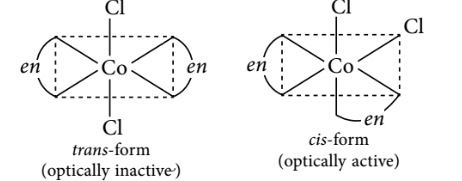Stereo isomerism
Question

# The type of isomerism shown by the complex $\left[{\mathrm{CoCl}}_{2}\left(en{\right)}_{2}\right]$ is

Moderate
Solution

## $\left[{\mathrm{CoCl}}_{2}\left(en{\right)}_{2}\right],$ exhibit geometrical isomerism, as the coordination number of Co is 6 and this compound has octahedral geometry.Get Instant Solutions